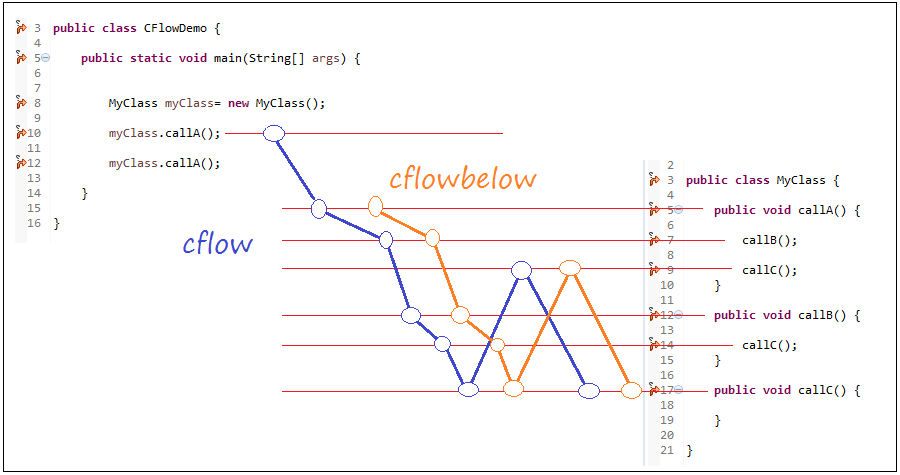# Java Aspect Oriented Programming Tutorial with AspectJ (AOP)

## 1- Introduction

This document is based on:

## 2- The required before start

You need to install the AspectJ development tools into Eclipse, you can see the instructions at:

## 3- The class participated in the example

In this document, I use some classes that participate in many illustrated examples of AspectJ.
• Box
• FigureElement
• Group
• Line
• Point
• ShapeFigureElement
• SlotfulPoint
The source of these classes is from: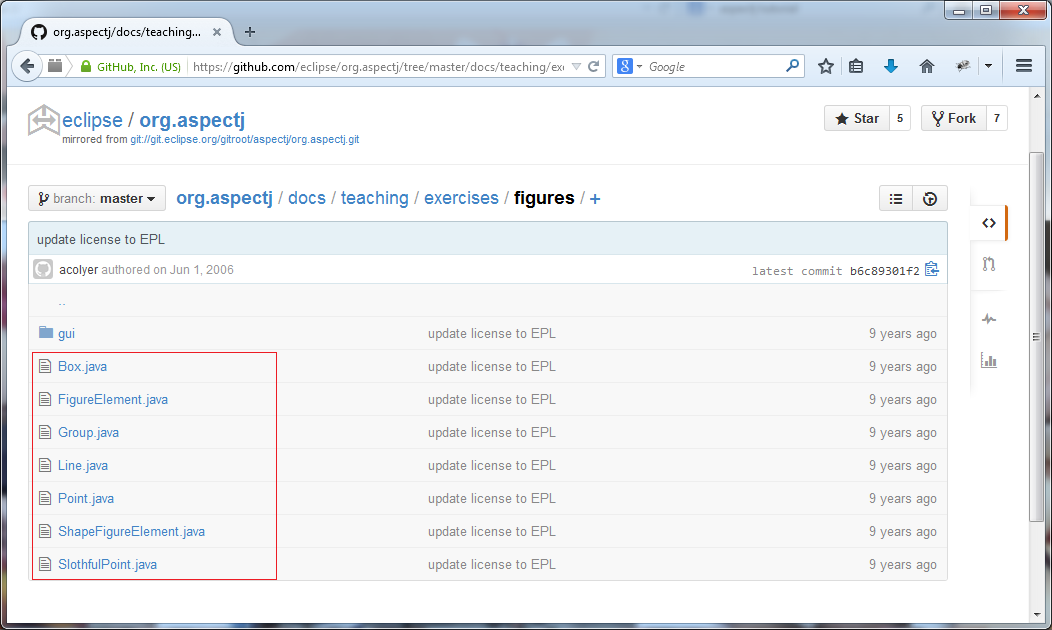Box.java
```package figures;

import java.awt.Rectangle;
import java.awt.Shape;

public class Box extends ShapeFigureElement {
private Point _p0;
private Point _p1;
private Point _p2;
private Point _p3;

public Box(int x0, int y0, int width, int height) {
_p0 = new Point(x0, y0);
_p1 = new Point(x0 + width, y0);
_p2 = new Point(x0 + width, y0 + height);
_p3 = new Point(x0, y0 + height);
}

public Point getP0() {
return _p0;
}

public Point getP1() {
return _p1;
}

public Point getP2() {
return _p2;
}

public Point getP3() {
return _p3;
}

@Override
public void move(int dx, int dy) {
_p0.move(dx, dy);
_p1.move(dx, dy);
_p2.move(dx, dy);
_p3.move(dx, dy);
}

public void checkBoxness() {
if ((_p0.getX() == _p3.getX()) && (_p1.getX() == _p2.getX())
&& (_p0.getY() == _p1.getY()) && (_p2.getY() == _p3.getY()))
return;
throw new IllegalStateException("This is not a square.");
}

@Override
public String toString() {
return "Box(" + _p0 + ", " + _p1 + ", " + _p2 + ", " + _p3 + ")";
}

@Override
public Shape getShape() {
return new Rectangle(getP1().getX(), getP1().getY(), getP3().getX()
- getP1().getX(), getP3().getY() - getP1().getY());
}
}
```
FigureElement.java
```package figures;

import java.awt.*;
import java.awt.geom.*;

public interface FigureElement {
public static final Rectangle MAX_BOUNDS = new Rectangle(0, 0, 500, 500);

public abstract void move(int dx, int dy);

public abstract Rectangle getBounds();

public abstract boolean contains(Point2D p);

public abstract void paint(Graphics2D g2);
}
```
Group.java
```package figures;

import java.awt.Graphics2D;
import java.awt.Rectangle;
import java.awt.geom.Point2D;
import java.util.ArrayList;
import java.util.Collection;
import java.util.Iterator;

public class Group implements FigureElement {
private Collection _members;
private String _identifier;

public Group(FigureElement first) {
this._members = new ArrayList();
}

}

public Iterator members() {
return _members.iterator();
}

public void move(int dx, int dy) {
for (Iterator i = _members.iterator(); i.hasNext();) {
FigureElement fe = (FigureElement) i.next();
fe.move(dx, dy);
}
}

public void setIdentifier(String identifier) {
_identifier = identifier;
}

@Override
public String toString() {
if (_identifier != null) {
return _identifier;
}
StringBuffer buf = new StringBuffer("Group(");
for (Iterator i = _members.iterator(); i.hasNext();) {
buf.append(i.next().toString());
if (i.hasNext()) {
buf.append(", ");
}
}
buf.append(")");
return buf.toString();
}

public Rectangle getBounds() {
Rectangle previous = null;
for (Iterator i = _members.iterator(); i.hasNext();) {
FigureElement fe = (FigureElement) i.next();
Rectangle rect = fe.getBounds();
if (previous != null) {
previous = previous.union(rect);
} else {
previous = rect;
}
}
return previous;
}

public boolean contains(Point2D p) {
for (Iterator i = _members.iterator(); i.hasNext();) {
FigureElement fe = (FigureElement) i.next();
if (fe.contains(p))
return true;
}
return false;
}

public void paint(Graphics2D g2) {
for (Iterator i = _members.iterator(); i.hasNext();) {
FigureElement fe = (FigureElement) i.next();
fe.paint(g2);
}
}

public int size() {
return _members.size();
}
}
```
Line.java
```package figures;

import java.awt.*;
import java.awt.geom.*;

public class Line extends ShapeFigureElement {
private Point _p1;
private Point _p2;

public Line(Point p1, Point p2) {
_p1 = p1;
_p2 = p2;
}

public Point getP1() {
return _p1;
}

public Point getP2() {
return _p2;
}

@Override
public void move(int dx, int dy) {
_p1.move(dx, dy);
_p2.move(dx, dy);
}

@Override
public String toString() {
return "Line(" + _p1 + ", " + _p2 + ")";
}

/**
* Used to determine if this line {@link contains(Point2D)} a point.
*/
final static int THRESHHOLD = 5;

/**
* Returns <code>true</code> if the point segment distance is less than
*/
@Override
public boolean contains(Point2D p) {
return getLine2D().ptLineDist(p) < THRESHHOLD;
}

private Line2D getLine2D() {
return new Line2D.Float((float) getP1().getX(), (float) getP1().getY(),
(float) getP2().getX(), (float) getP2().getY());
}

public Shape getShape() {
return getLine2D();
}
}
```
Point.java
```package figures;

import java.awt.*;
import java.awt.geom.*;

public class Point extends ShapeFigureElement {
private int _x;
private int _y;

public Point(int x, int y) {
_x = x;
_y = y;
}

public int getX() {
return _x;
}

public int getY() {
return _y;
}

public void setX(int x) {
_x = x;
}

public void setY(int y) {
_y = y;
}

@Override
public void move(int dx, int dy) {
_x += dx;
_y += dy;
}

@Override
public String toString() {
return "Point(" + _x + ", " + _y + ")";
}

/** The height of displayed {@link Point}s. */
private final static int HEIGHT = 10;
/** The width of displayed {@link Point}s. -- same as {@link HEIGHT}. */
private final static int WIDTH = Point.HEIGHT;

@Override
public Shape getShape() {
return new Ellipse2D.Float((float) getX() - Point.WIDTH / 2,
(float) getY() - Point.HEIGHT / 2, (float) Point.HEIGHT,
(float) Point.WIDTH);
}
}
```
ShapeFigureElement.java
```package figures;

import java.awt.*;
import java.awt.geom.*;

public abstract class ShapeFigureElement implements FigureElement {
@Override
public abstract void move(int dx, int dy);

public abstract Shape getShape();

@Override
public Rectangle getBounds() {
return getShape().getBounds();
}

@Override
public boolean contains(Point2D p) {
return getShape().contains(p);
}

public Color getLineColor() {
return Color.black;
}

public Color getFillColor() {
return Color.red;
}

@Override
public final void paint(Graphics2D g2) {
Shape shape = getShape();
g2.setPaint(getFillColor());
g2.fill(shape);
g2.setPaint(getLineColor());
g2.draw(shape);
}
}
```
SlothfulPoint.java
```package figures;

import java.awt.*;
import java.awt.geom.*;

/**
* This class makes mistakes to be caught by invariant checkers.
*/
public class SlothfulPoint extends ShapeFigureElement {
private int _x;
private int _y;

public SlothfulPoint(int x, int y) {
}

public int getX() {
return _x;
}

public int getY() {
return _y;
}

public void setX(int x) {
}

public void setY(int y) {
}

@Override
public void move(int dx, int dy) {
System.out.println("Slothful moving");
}

@Override
public String toString() {
return "SlothfulPoint";
}

@Override
public Shape getShape() {
return new Ellipse2D.Float((float) _x, (float) _y, 1.0f, 1.0f);
}
}
```

## 4- Create AspectJ Project

First create a common Project named AspectJTutorial.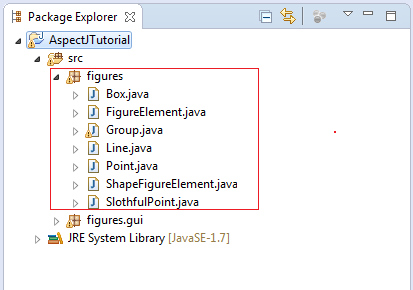Right-click Project, select Configure/Convert to AspectJ Project.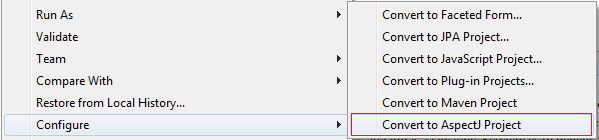Convert success: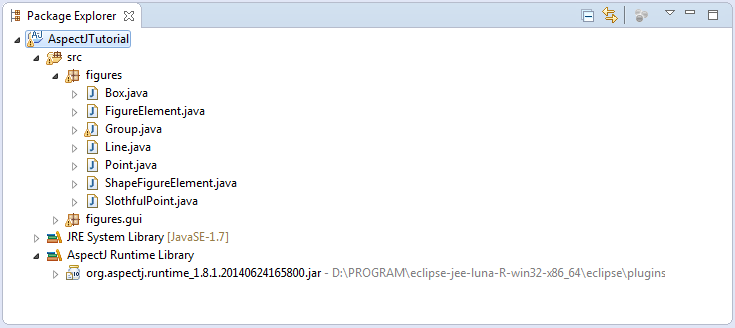## 5- First example with AspectJ

First, we practice the first example before starting with concepts:
Create class HelloAspectJDemo:
HelloAspectJDemo.java
```package org.o7planning.tutorial.aspectj.helloaspectj;

public class HelloAspectJDemo {

public static void sayHello() {
System.out.println("Hello");
}

public static void greeting()  {

String name = new String("John");

sayHello();

System.out.print(name);
}

public static void main(String[] args) {

sayHello();

System.out.println("--------");

sayHello();

System.out.println("--------");

greeting();

}

}
```
Such an above class is unnoticeable. However, the problem is that you want the program to do something right before or after sayHello() method is called. For example, printing a message. Traditionally, you can insert code printing a message right before or after you call sayHello() method.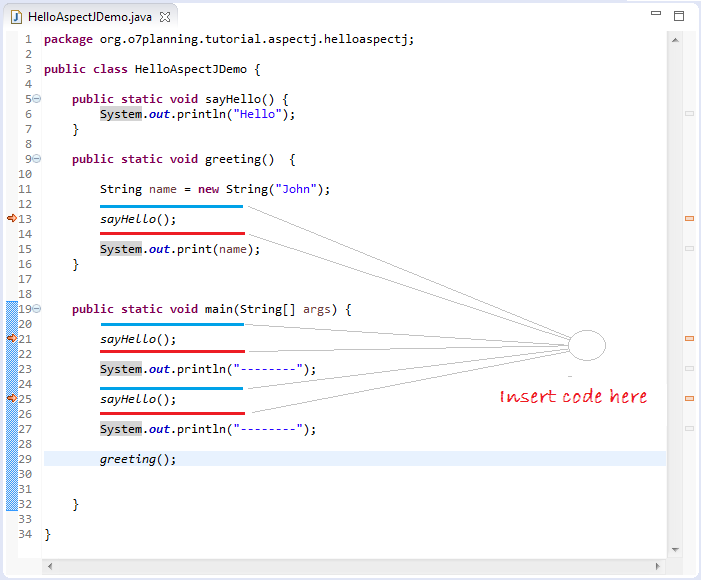AspectJ is a kind of aspect programming. It provides you with another solution to solve this problem.
• File/New/Other..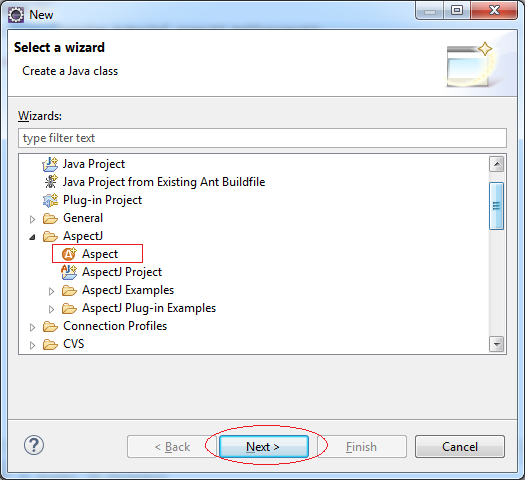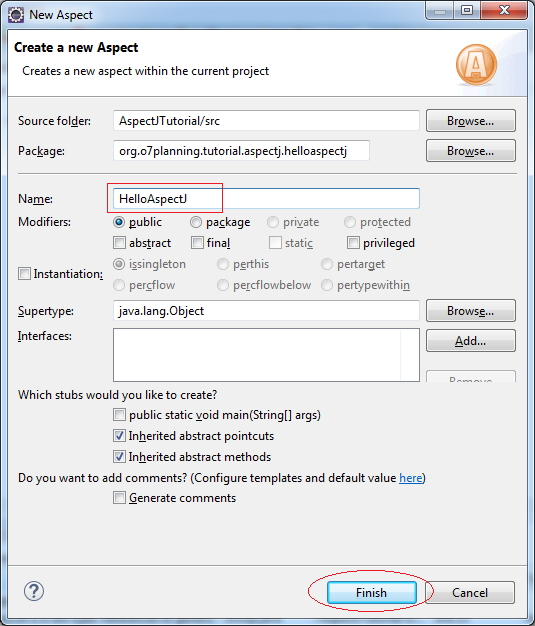A file named HelloAspectJ.aj is created, and its structure is quite alike a class's.
The previous versions of AspectJ use Annotation for description. The recent versions use *.aj file. In this document, I skip the use of Annotation since it is not as obvious as that of *.aj file. Besides, the syntax of *aj file is alike a class's, and eclipse will notify you of your syntax errors.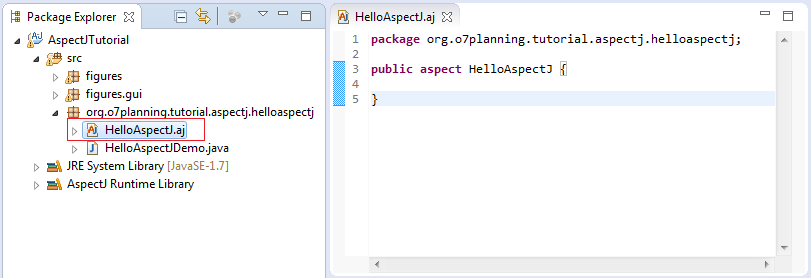Edit code of HelloAspectJ.aj:
HelloAspectJ.aj
```package org.o7planning.tutorial.aspectj.helloaspectj;

public aspect HelloAspectJ {

// Define a Pointcut is
// collection of JoinPoint call sayHello of class HelloAspectJDemo.
pointcut callSayHello(): call(* HelloAspectJDemo.sayHello());

before() : callSayHello() {
System.out.println("Before call sayHello");
}

after() : callSayHello()  {
System.out.println("After call sayHello");
}

}
```
AspectJ has some differences from a typical class in which it adds many keywords. Its file name has the tail of *.aj instead of *.java. Some keywords of AspectJ:
• aspect   pointcut   privileged   call   execution
• initialization   preinitialization   handler   get   set
• staticinitialization   target   args   within   withincode
• cflow   cflowbelow   annotation   before   after   around
• proceed   throwing   returning   adviceexecution   declare
• parents   warning   error   soft   precedence   thisJoinPoint
• thisJoinPointStaticPart   thisEnclosingJoinPointStaticPart
• issingleton   perthis   pertarget   percflow   percflowbelow
• pertypewithin   lock   unlock   thisAspectInstance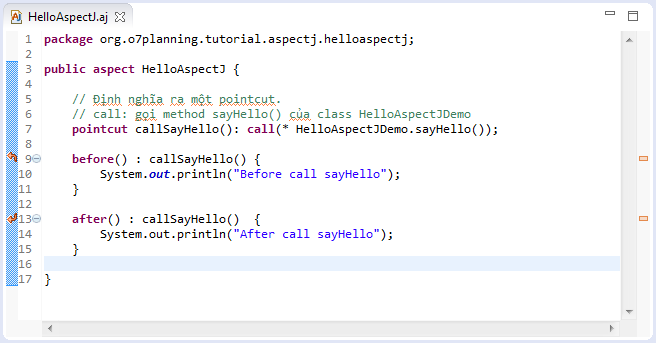Turn back to the example of HelloWorld. Now we run  HelloAspectJDemo class, and the returning result is: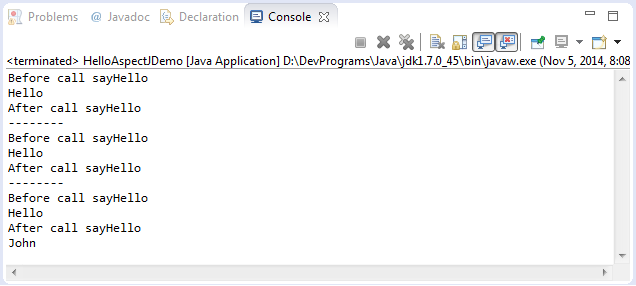AspectJ is very strong. For example, in the above example, correcting the code on HelloAspectJ.aj can make it work well with every class calling sayHello() method.
```// Define a pointcut is a Collection of JoinPoint
// call sayHello() of HelloAspectJDemo
pointcut callSayHello(): call(* HelloAspectJDemo.sayHello());

// Change to:
// This means that everywhere (class, AspectJ) called sayHello()
pointcut callSayHello(): call(* *.sayHello());
```

## 6- The basic concepts of AspectJ

In AspectJ you need to distinguish several concepts:
• Pointcut
• JoinPoint

### 6.1- JoinPoint

A join point is a well-defined point in the program flow
• We want to execute some code (“advice”) each time a join point is reached
• We do not want to clutter up the code with explicit indicators saying “This is a join point”
• AspectJ provides a syntax for indicating these join points “from outside” the actual code
A join point is a point in the program flow “where something happens”
• When a method is called
• When an exception is thrown
• When a variable is accessed
• When instantiating an object
• When referring an object
Thus JoinPoint is very diverse, where you initialize an object is also considered a JoinPoint.
Back to the HelloWorld example we will specify JoinPoints: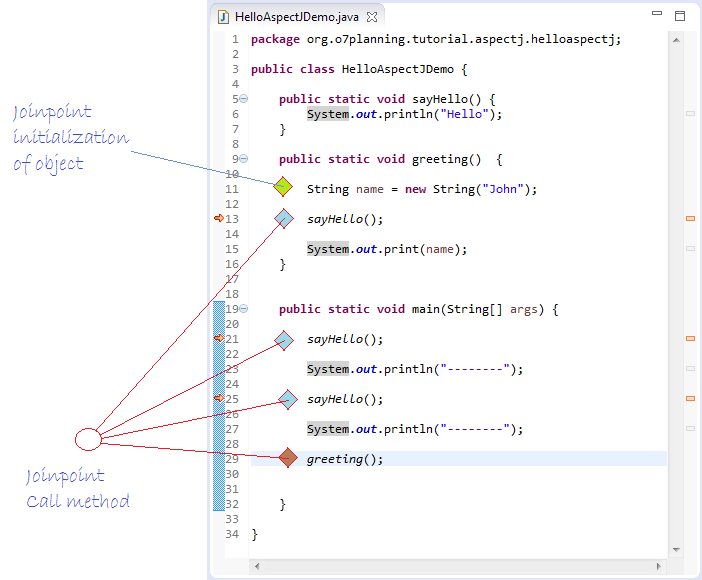### 6.2- PointCut

Pointcut definitions consist of a left-hand side and a right-hand side, separated by a colon
• The left-hand side consists of the pointcut name and the pointcut parameters (i.e. the data available when the events happen)
• The right-hand side consists of the pointcut itself
Example:
```pointcut callSayHello(): call(* HelloAspectJDemo.sayHello());
```
• The name of this pointcut is callSayHello
• The pointcut has no parameters
• The pointcut itself is call(* HelloAspectJDemo.sayHello())
• The pointcut refers to any time the HelloAspectJDemo.sayHello() method is called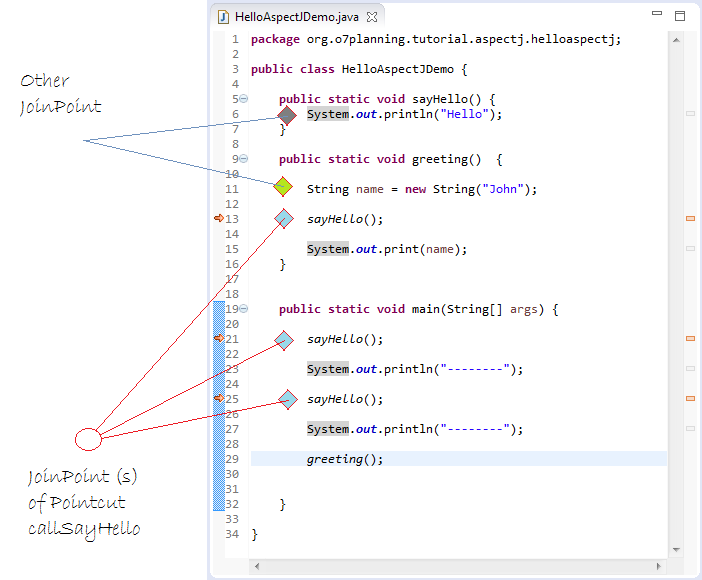Back to the HelloWorld example, we have two Advice
• before()
• after()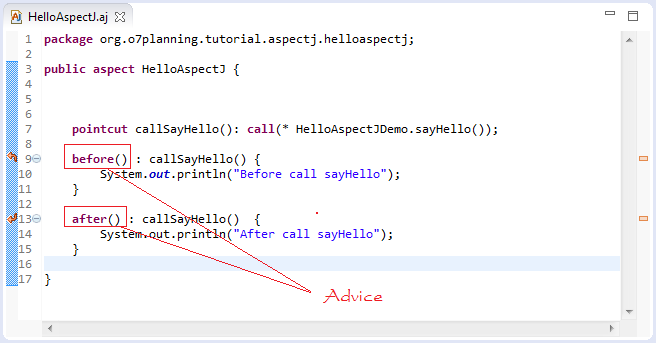Advice defines crosscutting behavior. It is defined in terms of pointcuts. The code of a piece of advice runs at every join point picked out by its pointcut. Exactly how the code runs depends on the kind of advice.

AspectJ supports three kinds of advice. The kind of advice determines how it interacts with the join points it is defined over. Thus AspectJ divides advice into that which runs before its join points, that which runs after its join points, and that which runs in place of (or "around") its join points.

While before advice is relatively unproblematic, there can be three interpretations of after advice: After the execution of a join point completes normally, after it throws an exception, or after it does either one. AspectJ allows after advice for any of these situations.
For ease of understanding we see a few examples with Advice:
HelloAspectJ2.aj
```package org.o7planning.tutorial.aspectj.helloaspectj;

public aspect HelloAspectJ2 {

pointcut callSayHello(): call(* HelloAspectJDemo.sayHello());

after() returning (Object retObj): callSayHello() {
System.out.println("Returned normally with " + retObj);
}

after() throwing (Exception e): callSayHello() {
System.out.println("Threw an exception: " + e);
}

// Advice "after returning" + "after throwing".
after() : callSayHello()  {
System.out.println("Returned or threw an Exception");
}
}
```
Advice  "after returning" may not care about the returning value of method, so we can write it down..
```// Advice "after returning".
// care about the returning value of method
after() returning (Object retObj): callSayHello() {
System.out.println("Returned normally with " + retObj);
}

// Advice "after returning" not care about the returning value of method
after() returning(): callSayHello() {
System.out.println("Returned normally");
}

// or
// Advice "after returning" not care about the returning value of method
after() returning : callSayHello() {
System.out.println("Returned normally");
}
```

## 7- Basic AspectJ examples

Before moving on advanced details, we see basic examples and rules in AspectJ. Understanding these rules is a prerequisite for understanding AspectJ.

### 7.1- Rules for class, method, * and ..

In AspectJ, if you want to refer to a class or a method in declaring Pointcut, you will have to write down its fully qualified name. For example:
• figures.Point
• figures.Point.setX(int)
If class and AspectJ are in the same package, you can write briefly. For example:
• Point
• Point.setX(int)
Let see a following illustration,  AspectJ01.aj and class  Point are in two different packages.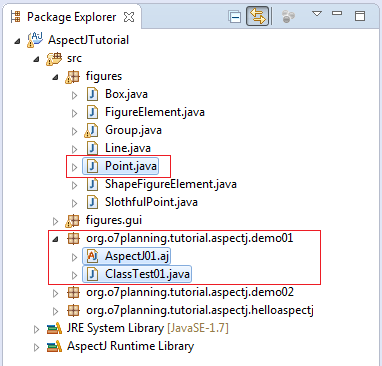AspectJ01.aj
```package org.o7planning.tutorial.aspectj.demo01;

public aspect AspectJ01 {

// Class Point and AspectJ is not the same package
// so must specify the package (Required).
// This pointcut definition of JoinPoints
// only within the class ClassTest01
// This ClassTest01 and AspectJ same package,
// so can be ignored package in within(..).
pointcut callSetX()  : call(void  figures.Point.setX(int)) && within (ClassTest01) ;

before() : callSetX()  {
System.out.println("Before call Point.setX(int)");
}
}
```
ClassTest01.java
```package org.o7planning.tutorial.aspectj.demo01;

import figures.Point;

public class ClassTest01 {

public static void main(String[] args) {

Point point = new Point(10, 200);

System.out.println("---- (1) ----");

point.setX(20);

System.out.println("---- (2) ----");

point.setY(100);

System.out.println("---- (3) ----");
}

}
```
Results of running ClassTest01: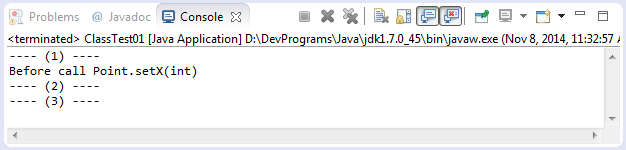So here there are some notes:
```// 'within' used to limit the scope of the pointcut
// in the case below:
// Only Containing join point inside class ClassTest01.
within (ClassTest01)

// If you using import
import figures.Point;
.....
// Then Can write shorter code:
pointcut callSetX()  : call(void  Point.setX(int)) && within (ClassTest01) ;
```
The asterisk (*) in AspectJ
```// Collection of JoinPoints call Point.setX(int), any package name,
// and method return  void
pointcut callSetX()  : call(void  *.Point.setX(int)) ;

// Collection of JoinPoints call Point.setX(int), any package name,
// and method return any
pointcut callSetX()  : call(* *.Point.setX(int)) ;

// Collection of JoinPoints call public static method setX(int) of class with package name is 'sample'
// and class name have suffix Point,
// and setX(int) return int
pointcut callSetX()  : call(public static int sample.*Point.setX(int)) ;

// Using (..) to describe the method has 0 or more parameters.
pointcut callSetX()  : call(public static int sample.*Point.setX(..)) ;
```

### 7.2- Target & args

target: That is the Java object on which the method is called (or the object whose field is accessed).
args: Arguments

See a Example:

AspectJ02.aj
```package org.o7planning.tutorial.aspectj.demo02;

import figures.Point;

public aspect AspectJ02 {

// Using target: define object on which the method is called
// Using args: define args on the method is called
// Using within: to restrict JoinPoint within ClassTest02
pointcut callMove(Point point, int dx, int dy)
: call(*  figures.Point.move(int,int))
&& args(dx,dy) && target(point) && within(ClassTest02)  ;

before(Point point, int dx, int dy) : callMove(point,  dx, dy )  {
System.out.println("Before call move(" + dx + "," + dy + ")");
System.out.println(point.toString());
}
}
```
ClassTest02.java
```package org.o7planning.tutorial.aspectj.demo02;

import figures.Point;

public class ClassTest02 {

public static void main(String[] args) {

Point point = new Point(10, 200);

System.out.println("---- (1) ----");

point.move(20, 30);

System.out.println("---- (2) ----");

System.out.println(point.toString());

System.out.println("---- (3) ----");

point.setX(100);
}

}
```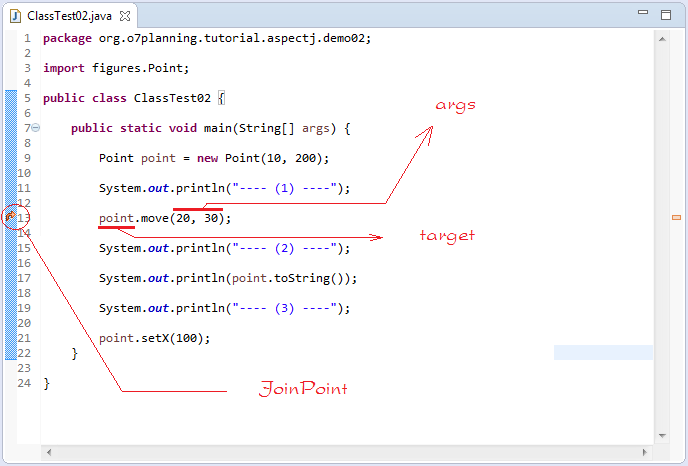Results of running the example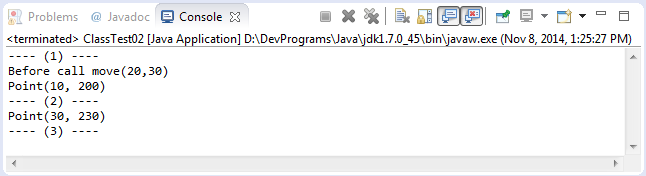### 7.3- Operator && ||

AspectJ03.aj
```package org.o7planning.tutorial.aspectj.demo03;

// Note: Must import FigureElement & Point
import figures.FigureElement;
import figures.Point;

public aspect AspectJ03 {

// pointcut: Include move actions
pointcut moveAction() :  (
call(void FigureElement.move(int,int)) ||
call(void Point.setX(int))              ||
call(void Point.setY(int))
)
&& within (ClassTest03);

before() : moveAction()  {
System.out.println("before move");
}

}
```
ClassTest03.java
```package org.o7planning.tutorial.aspectj.demo03;

import figures.FigureElement;
import figures.Line;
import figures.Point;

public class ClassTest03 {
public static void main(String[] args) {

Point point = new Point(10, 200);

System.out.println("---- (1) ----");

point.setX(20 );

System.out.println("---- (2) ----");

FigureElement line= new Line(new Point(1,1), new Point(10,10));

line.move(10, 10);

System.out.println("---- (3) ----");
}
}
```
Results of running the example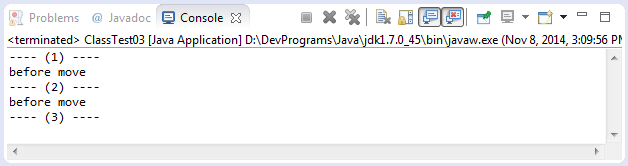### 7.4- Field in AspectJ

FieldInAspectJ.aj
```package org.o7planning.tutorial.aspectj.demo04;

import java.io.PrintStream;

public aspect FieldInAspectJ {

// Field in AspectJ.
PrintStream logStream = System.err;

pointcut move() : call(* figures.Point.move(int,int)) && within(FieldInAspectJTest);

before(): move() {
logStream.println("Before Point move");
}
}
```
FieldInAspectJTest.java
```package org.o7planning.tutorial.aspectj.demo04;

import figures.Point;

public class FieldInAspectJTest {

public static void main(String[] args) {

Point point = new Point(10, 200);

System.err.println("---- (1) ----");

point.setX(20);

System.err.println("---- (2) ----");

point.move(10, 10);

System.err.println("---- (3) ----");
}
}
```
Results of running the example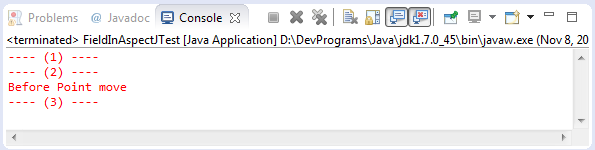### 7.5- Inter-type declarations

PointObserving.aj
```package org.o7planning.tutorial.aspectj.demo05;

import java.util.ArrayList;
import java.util.List;

import figures.Point;

public aspect PointObserving {

// Class Point have no field: observers
// However, it can declare here.
// observers: Store the change point position.
private List<Point> Point.observers = new ArrayList<Point>();

pointcut moveAction(Point point) : call(void Point.move(int,int) )
&& target(point)
&& within(PointObservingTest);

after(Point point) : moveAction(point)  {
System.out.println("Point moved");

// Print the location of the point went through.
System.out.println(" - "+point.observers);
}

public static void addObserver(Point p) {
}

public static void removeObserver(Point p) {
// p.observers.remove(s);
}
}
```
PointObservingTest.java
```package org.o7planning.tutorial.aspectj.demo05;

import figures.Point;

public class PointObservingTest {

public static void main(String[] args) {

Point point1 = new Point(100, 100);

// First move
point1.move(10, 10);

// Second move
point1.move(10, 10);

System.out.println("----------------------");

Point point2 = new Point(200, 200);

// First move
point2.move(15, 10);

// Second move
point2.move(15, 10);

// Third Move
point2.move(25, 10);
}
}
```
Results of running the example: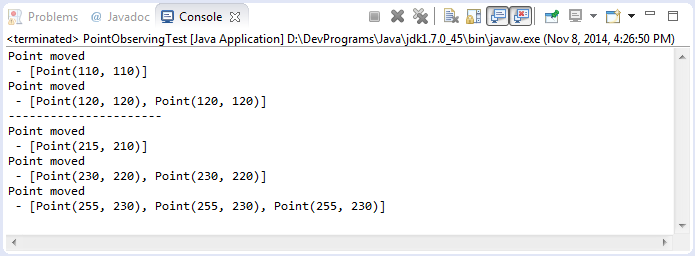### 7.6- Tracking

Method tracking:
We’ll define an Aspect that defines a pointcut on every method execution, as well as some advice to run when they turn up when the code is executed.
SimpleTracing.aj
```package org.o7planning.tutorial.aspectj.demo06;

import org.aspectj.lang.Signature;

public aspect SimpleTracing {

// Collection of JoinPoint call any method
// And within SimpleTracingTest
pointcut tracedCall() : call (* *(..))
//  && !within(SimpleTracing)
&& within(SimpleTracingTest)
;

before() : tracedCall()  {
Signature sig = thisJoinPointStaticPart.getSignature();
String line = ""
+ thisJoinPointStaticPart.getSourceLocation().getLine();

String sourceName = thisJoinPointStaticPart.getSourceLocation()
.getWithinType().getCanonicalName();
//
System.out.println("Call from " + sourceName + " line " + line + "\n   to "
+ sig.getDeclaringTypeName() + "." + sig.getName() +"\n");
}

}
```
SimpleTracingTest.java
```package org.o7planning.tutorial.aspectj.demo06;

import figures.Point;

public class SimpleTracingTest {

private static void testMethod1() {
Point point = new Point(100, 100);
point.setX(100);
}

private static void testMethod2() {

String text = "This is text";

String s = text.substring(2);

System.out.println(s);
}

public static void main(String[] args) {

testMethod1();

testMethod2();
}

}
```
Results of running the example: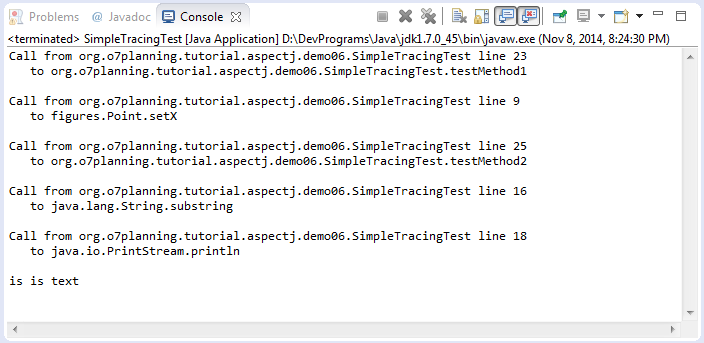### 7.7- Cflow

Control flow is just the flow of execution of program within specific join point. cflow() and cflowbelow() constructs takes another join point as argument and allow us to define control flow based pointcuts - the pointcuts that captures all the join points in the control flow of specified join point.
cflow() captures all the join points e.g. call, execution, set and get field, error handlers in the control flow of join point specified as argument along with the specified join point itself.
The illustration below contains the join point call MyClass.callA().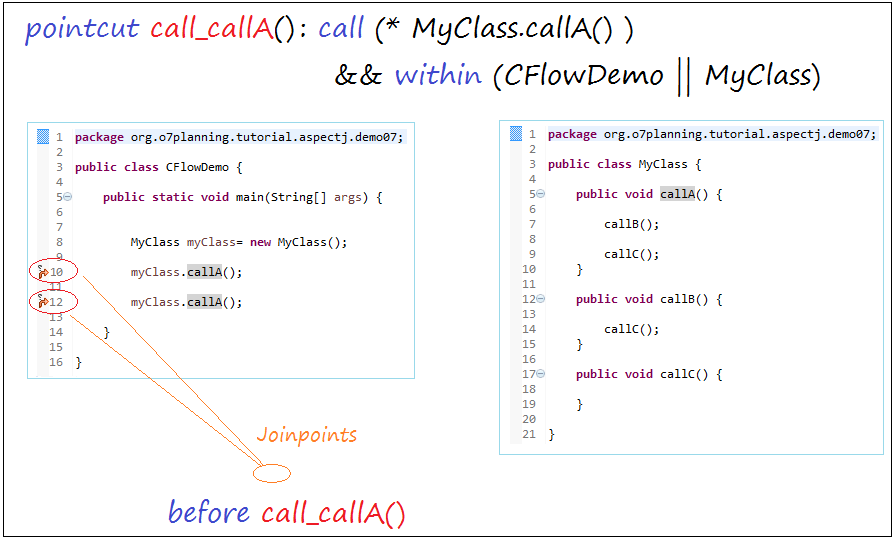Using cflow(..):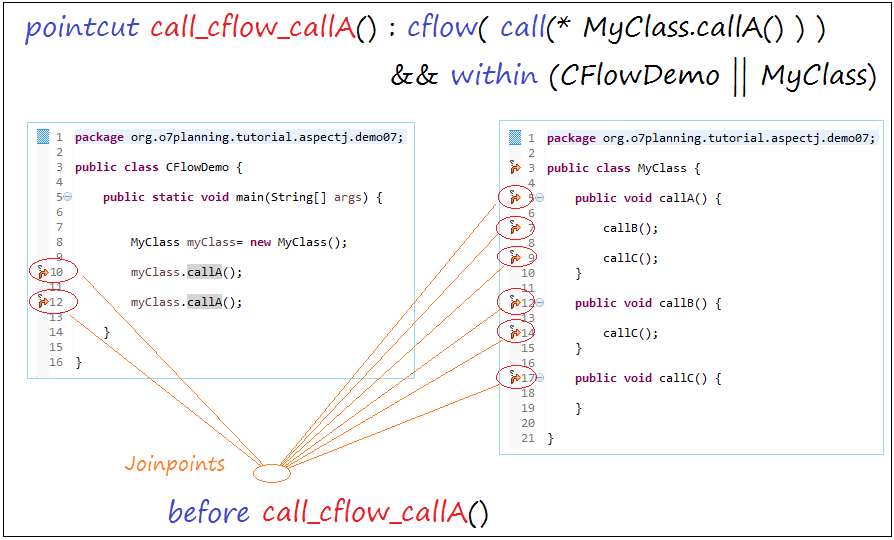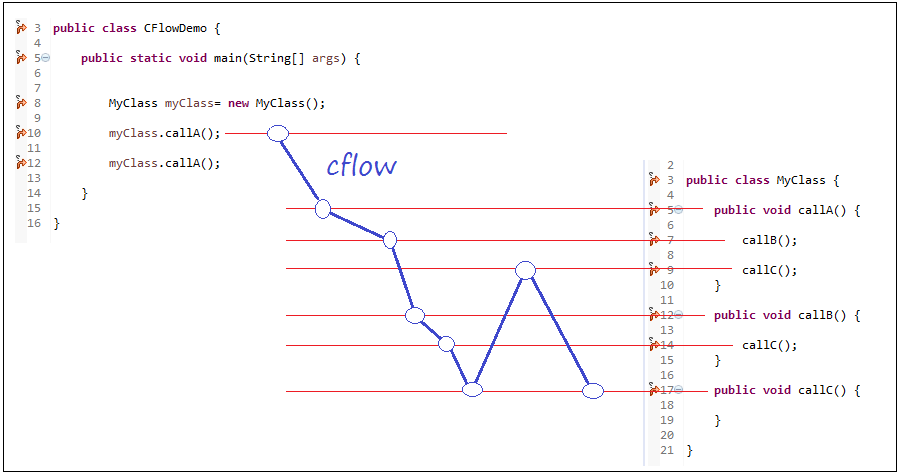See full example: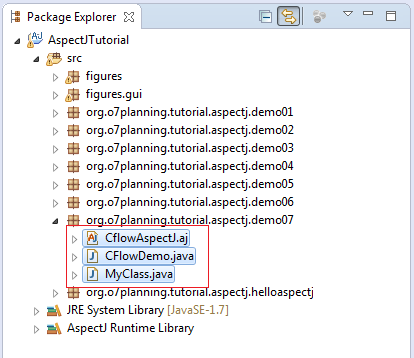CflowAspectJ.aj
```package org.o7planning.tutorial.aspectj.demo07;

public aspect CflowAspectJ {

pointcut call_cflow_callA() :  cflow( call( * MyClass.callA() ) )  && within(CFlowDemo || MyClass);

before() : call_cflow_callA()  {
System.out.println(
"Join Point at: " + thisJoinPointStaticPart.getSourceLocation().getWithinType().getCanonicalName()
+ " --> " + thisJoinPointStaticPart.getSourceLocation().getLine());
}

}
```
MyClass.java
```package org.o7planning.tutorial.aspectj.demo07;

public class MyClass {

public void callA() {

callB();

callC();
}

public void callB() {

callC();
}

public void callC() {

}

}
```
CFlowDemo.java
```package org.o7planning.tutorial.aspectj.demo07;

public class CFlowDemo {

public static void main(String[] args) {

MyClass myClass= new MyClass();

myClass.callA();

myClass.callA();

}

}
```
Results of running CFlowDemo class: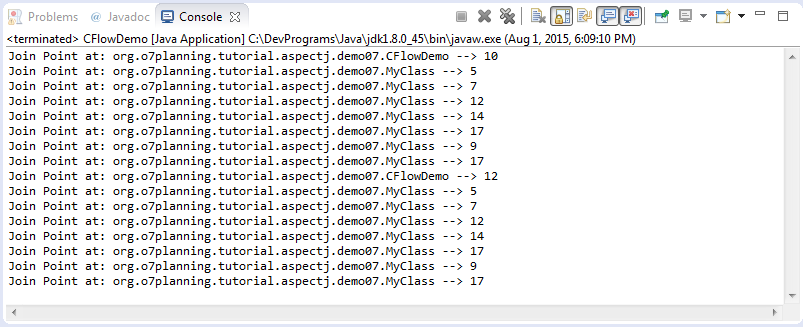### 7.8- Cflowbelow

Control flow is just the flow of execution of program within specific join point. cflow() and cflowbelow() constructs takes another join point as argument and allow us to define control flow based pointcuts - the pointcuts that captures all the join points in the control flow of specified join point.
cflowbelow() behaves same as cflow, however it doesnt captues the join point specified as argument, but captures all other join points that comes below that.
You can see more at the cflow() section.